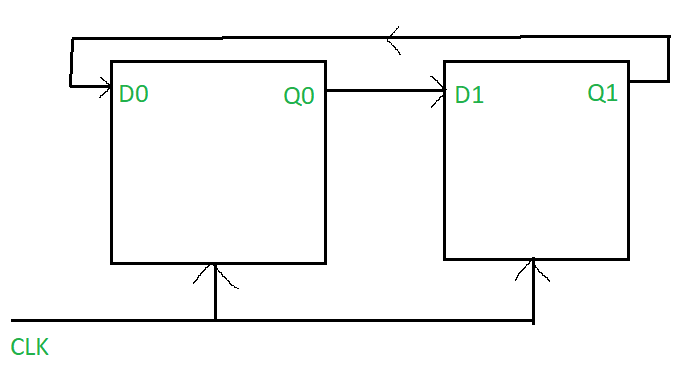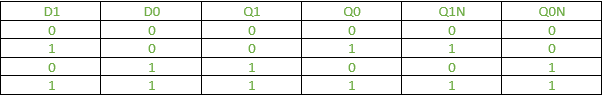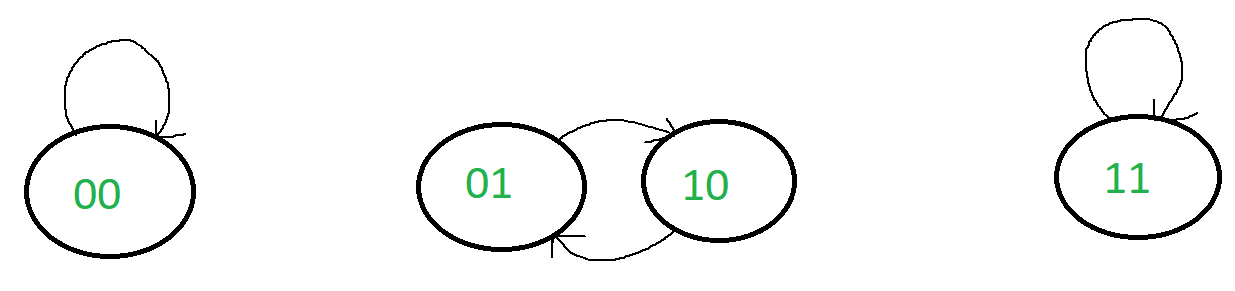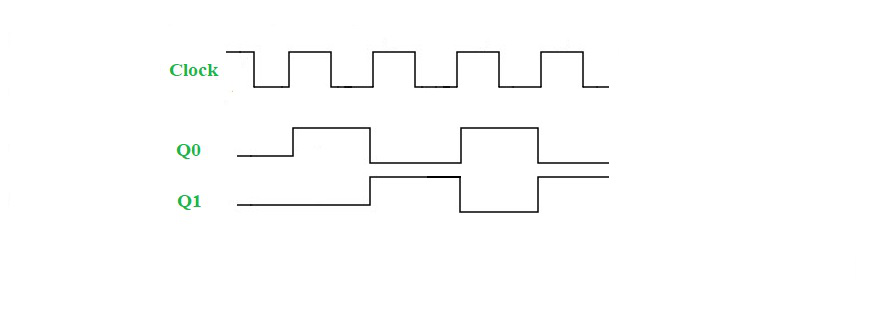# Mod 2 Ring Counter (with D flip-flop)

Ring counters are one of the most important applications of shift registers. They are created by connecting multiple flip-flops to one another (such that the output of one flip-flop is the input for another), and by connecting the output of the last flip-flop to the input of the first flip-flop.

For a mod 2-ring counter, two flip-flops will be required. Recall that the number of states that can be counted by a ring counter is equal to the number of flip-flops being used. Since a mod 2-ring counter can count up to 2 states, 2 flip-flops will be required. Since a ring counter is a synchronous counter, the clock needs to be in an “ON” state so that state transitions can happen and the ring counter can function normally.

### Circuit Diagram:From the diagram, we can see that D1 = Q0 and D0 = Q1

Table: Combining the excitation table and the state table here for convenience.### State Diagram:### Timing Diagram: